Function Repository Resource:

# ColoredNoise

Create an array with a specified slope in the power spectral density

Contributed by: Julien Kluge
 ResourceFunction["ColoredNoise"][count,alpha] creates array of length count with a slope of alpha/2 in the Fourier domain. ResourceFunction["ColoredNoise"][count,name] creates array of length count with a slope corresponding to the name noise color in the Fourier domain. ResourceFunction["ColoredNoise"][{c1,c2,…},alpha] creates a multidimensional array of lengths c1,c2, ….

## Details and Options

The mean of the created array is by default effectively zero.
The standard deviation of the created array is normalized to one.
The function threads over the alpha parameter.

## Examples

### Basic Examples (3)

Create 10 samples of white noise (slope zero):

 In:=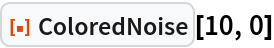Out=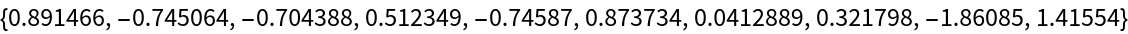Create 10 samples of pink noise (slope minus one):

 In:=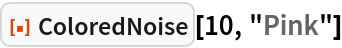Out=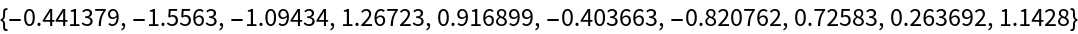Create violet noise in two dimensions:

 In:=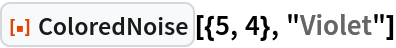Out=### Scope (3)

Plot different noise slopes in the time domain:

 In:=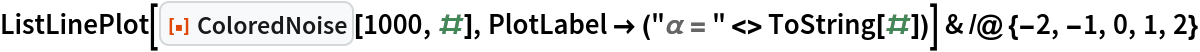Out=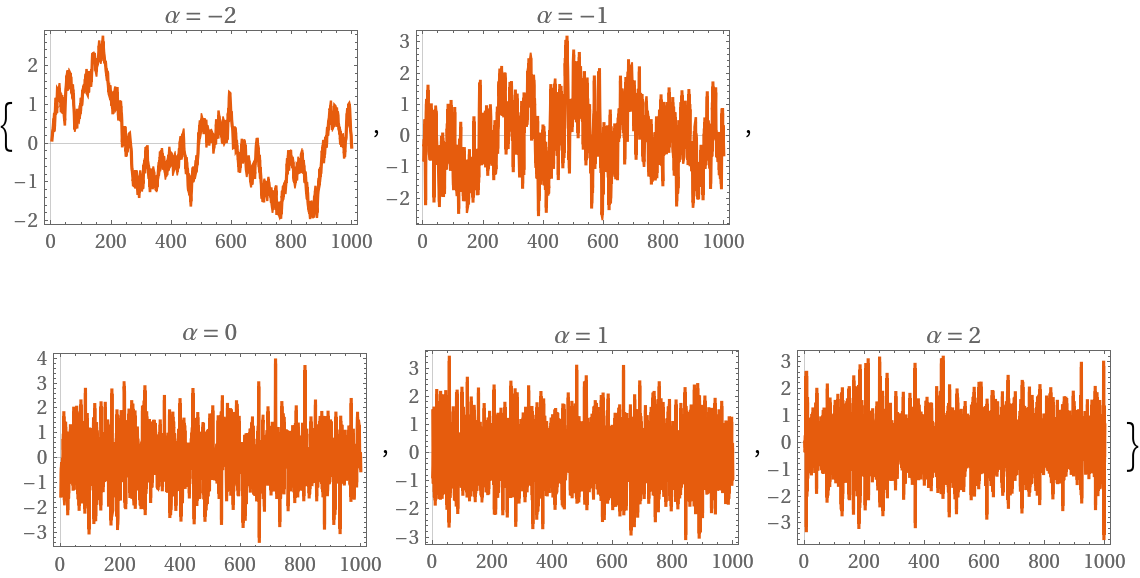Plot different noise slopes in the Fourier domain:

 In:=Out=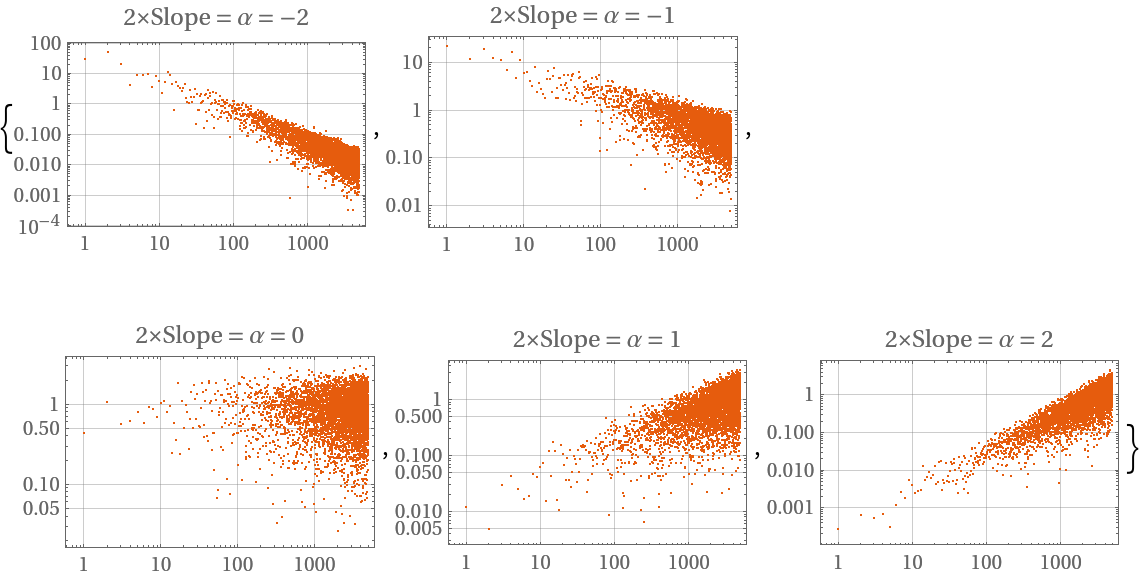Create the same display for two dimensions:

 In:=Out=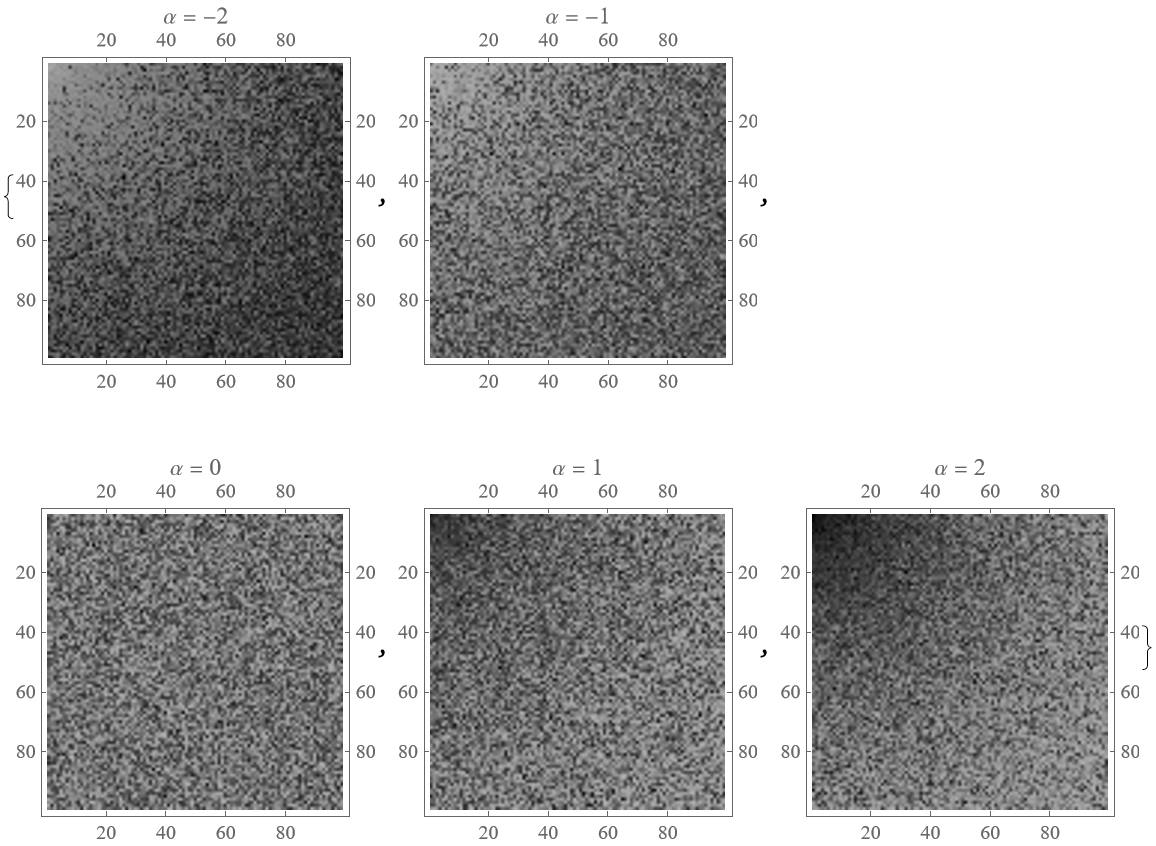All supported color keywords with their respective alpha applied:

 In:=Out=The mean and standard deviation are always zero and one, respectively:

 In:=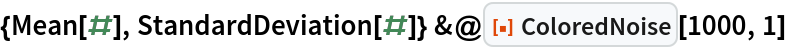Out=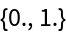### Options (4)

#### Deviation (2)

Turn off the normalization of the standard deviation:

 In:=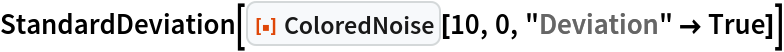Out=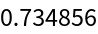Set the normalization of the standard deviation to a specific value:

 In:=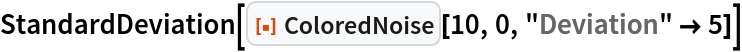Out=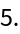#### FourierParameters (1)

Apply the Fourier transform with different FourierParameters:

 In:=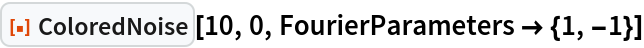Out=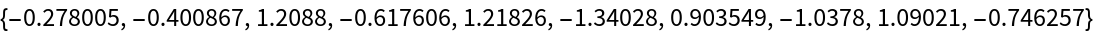#### Offset (1)

Set the offset to a specific value:

 In:=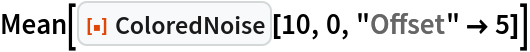Out=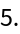### Applications (2)

Visualize the relation of alphas to the slopes in the power spectral density, the Allan deviation and the influence to the autocorrelation:

 In:=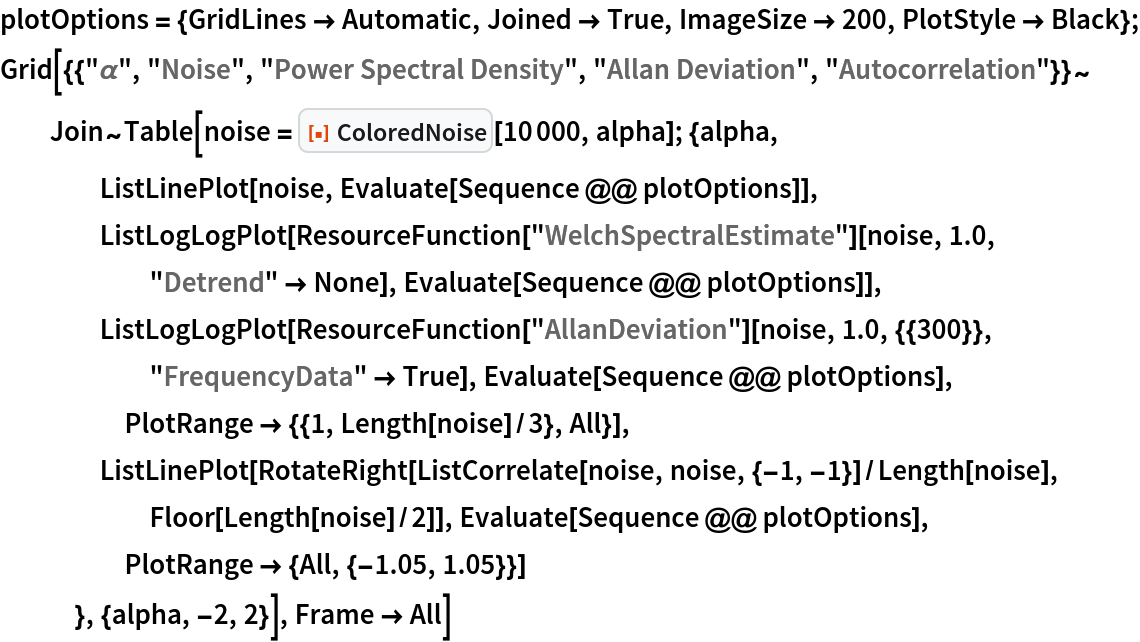Out=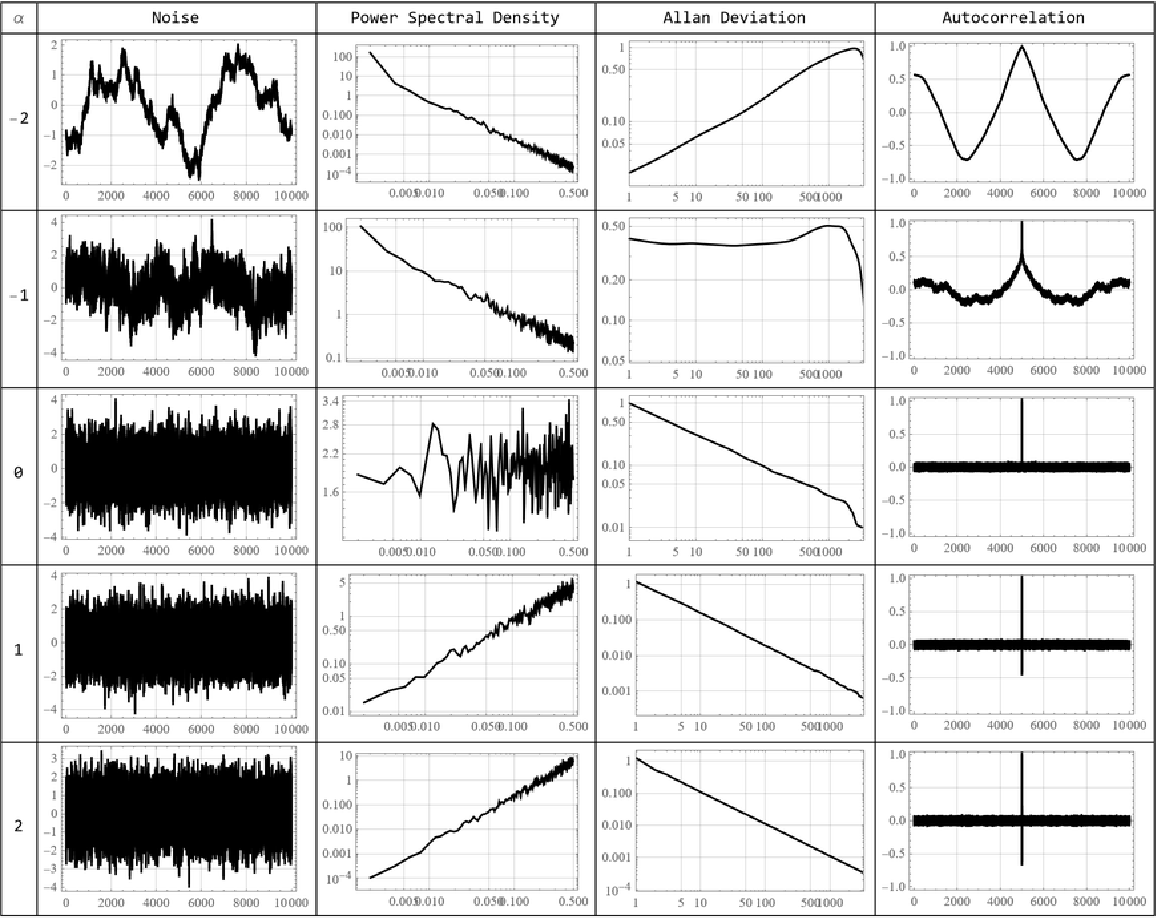Create array with a specified shape of the Allan deviation, mimicking an active atomic clock:

 In:=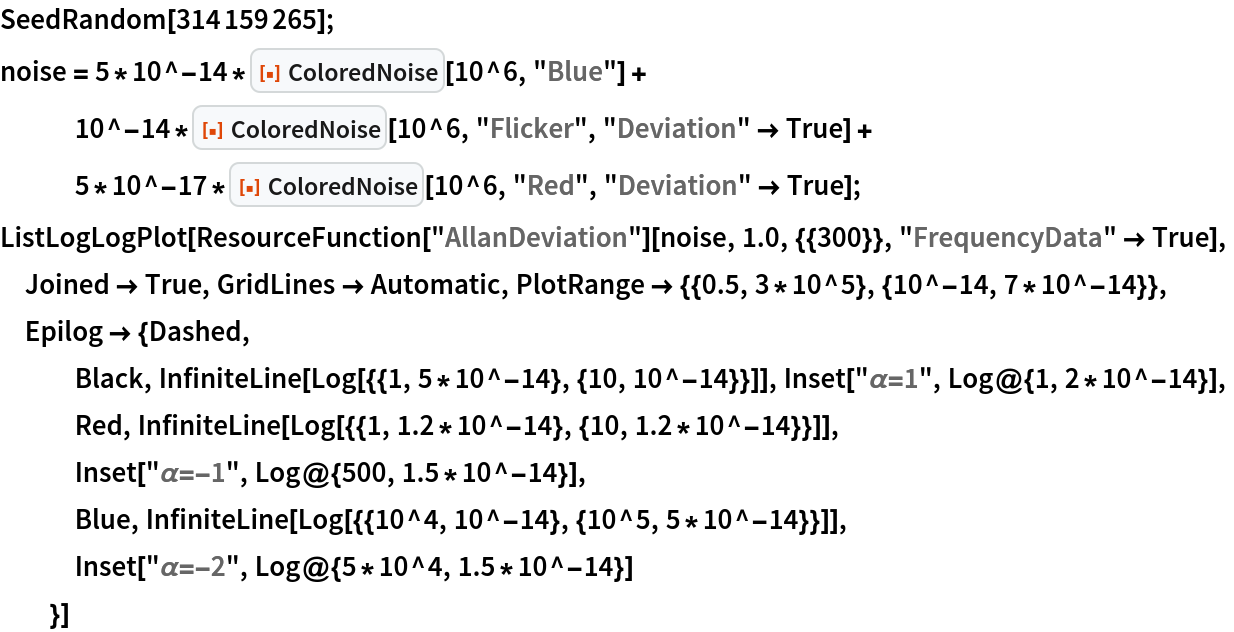Out=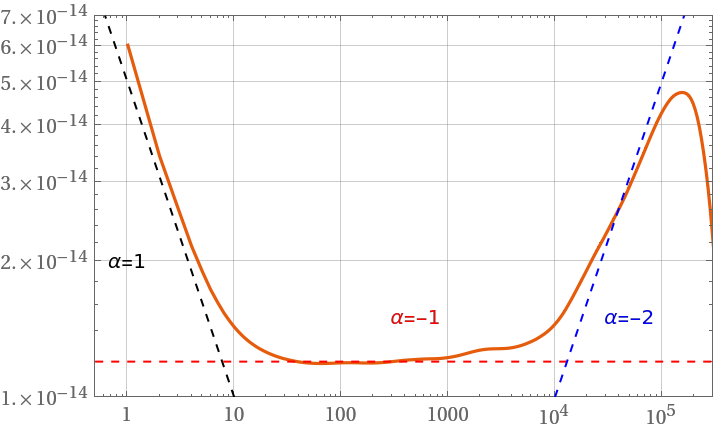### Possible Issues (2)

The samples argument must be greater than two:

 In:=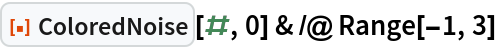Out=The alpha argument must be a known noise color or else a real number:

 In:=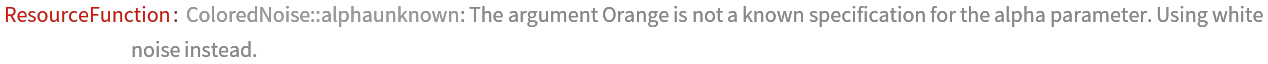Out=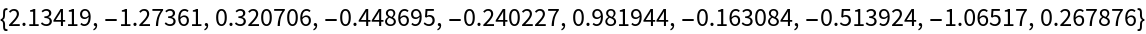### Neat Examples (2)

Noise with alpha values between -1 and -2 sound like a waterfall or turbulent flowing river:

 In:=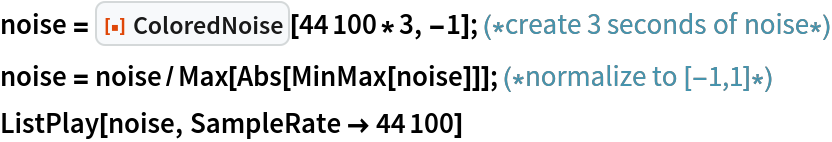Out=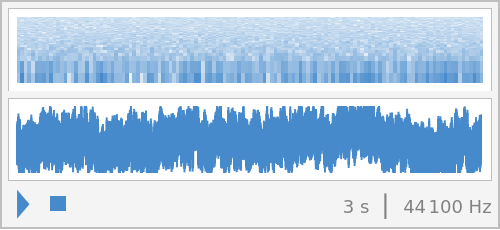Create soft 2D-noise maps by choosing low alpha values:

 In:=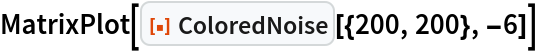Out=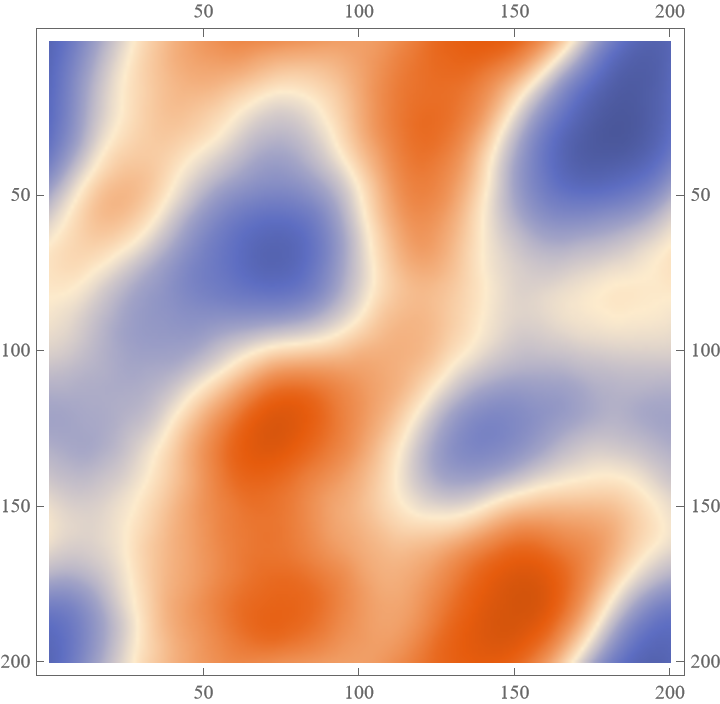Julien Kluge

## Version History

• 1.0.0 – 08 April 2022